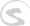Documents
Writing Docs Gigedit SFZ Instrument Scripts NKSP Language NKSP Reference

# max()

Returns the maximum value of the passed two value arguments. That means, it compares the two passed values with each other and returns the one which is larger.

### Function Prototype

`max(number1,number2) `

### Arguments

Argument Name Data Type Description
`number1` Integer Number or
Real Number
One of the two numbers to compare with.
[required]
`number2` Integer Number or
Real Number
One of the two numbers to compare with.
[required]

### Return Value

Data Type Description
Integer Number or
Real Number
Largest number of the passed two values.

### Remarks

This functions accepts any standard unit and finalness for its two arguments. However the unit types of the two arguments must be identical. The return value's unit type is identical to the two passed arguments' one, and the return value's finalness is the case if at least one of the two arguments has finalness.

### Examples

The following example prints the result of some example argument values to the terminal as soon as the script is loaded.

`min()`